# Glycolysis is the process by which energy is harvested from glucose by living things. Several of the reactions of glyco...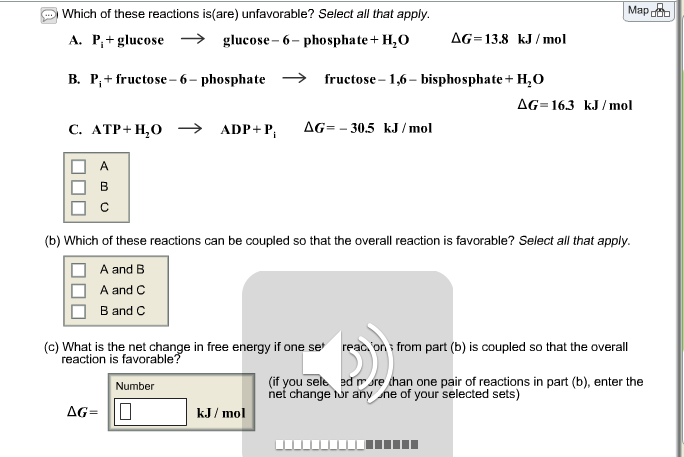Glycolysis is the process by which energy is harvested from glucose by living things. Several of the reactions of glycolysis are thermodynamically unfavorable (nonspontaneous), but proceed when they are coupled with other reactions.

Which of these reactions is (are) unfavorable? Select all that apply. Which of these reactions can be coupled so that overall reaction is favorable? Select all that apply. What is the net change in free energy if one selection from part (b) is coupled so that the overall reaction is favorable?

Concepts and reason

The problem is based on the concept of spontaneity of the reaction. For a reaction to be spontaneous, change in Gibbs free energy is less than zero. Gibbs free energy is related to enthalpy of the system as follows:

$\Delta G = \Delta H - T\Delta S$

Here, $\Delta H$ is the enthalpy change of the system, T is absolute temperature and $\Delta S$ is the entropy change of the system.

Fundamentals

For two reactions with different Gibbs free energy, the reaction with negative value of change in Gibbs free energy is spontaneous and favorable. If the value of Gibbs free energy is positive then the reaction is non-favorable.

(a)

Reactions showing glycolysis are as follows:

${P_i} + {\rm{glucose}} \to {\rm{glucose}} - 6 - {\rm{phosphate}} + {{\rm{H}}_{\rm{2}}}{\rm{O }}\Delta G = 13.8{\rm{ kJ mo}}{{\rm{l}}^{ - 1}}$ …… (A)

${P_i} + {\rm{fructose}} - 6 - {\rm{phosphate}} \to {\rm{fructose}} - 1,6 - {\rm{biphosphate}} + {{\rm{H}}_{\rm{2}}}{\rm{O }}\Delta G = 16.3{\rm{ kJ mo}}{{\rm{l}}^{ - 1}}$ …… (B)

${\rm{ATP}} + {{\rm{H}}_2}{\rm{O}} \to {\rm{ADP}} + {{\rm{P}}_i}{\rm{ }}\Delta {\rm{G}} = - 30.5{\rm{ kJ mo}}{{\rm{l}}^{ - 1}}$ …… (C)

For negative value of change in Gibbs free energy reactions are favorable. Therefore, those reaction which have positive value of change in Gibbs free energy are unfavorable. From above three reactions, the value of Gibbs free energy is positive for reaction (A) and reaction (B) therefore both the reactions are unfavorable.

(b)

Reactions are couples in such a way that final change in Gibbs free energy is negative. From reaction A, B and C, Since, value of Gibbs free energy is positive for both A and B the coupling of A and B will give positive value only.

Since, the value of Gibbs free energy is negative for only C whose magnitude is greater than Gibbs free energy of both A and B. Thus, the correct coupling is of reaction C with both A and B because for both the coupling, value of change in Gibbs free energy is negative means favorable reaction.

(c)

Since, the correct coupling is of reaction C with both A and B calculate change in Gibbs free energy of either of one set.

For A and C set,

Calculate net change in Gibbs free energy as follows:

$\Delta {G_{\rm{T}}} = \Delta {G_{\rm{A}}} + \Delta {G_{\rm{C}}}$

Substitute $13.8{\rm{ kJ mo}}{{\rm{l}}^{ - 1}}$ for $\Delta {G_{\rm{A}}}$ and $- 30.5{\rm{ kJ mo}}{{\rm{l}}^{ - 1}}$ for $\Delta {G_{\rm{C}}}$ thus,

$\begin{array}{c}\\\Delta {G_{\rm{T}}} = \left( {13.8{\rm{ kJ mo}}{{\rm{l}}^{ - 1}}} \right) + \left( { - 30.5{\rm{ kJ mo}}{{\rm{l}}^{ - 1}}} \right)\\\\ = - 16.7{\rm{ kJ mo}}{{\rm{l}}^{ - 1}}\\\end{array}$

Ans: Part a

Reaction A and B are unfavorable.

Part b

Reaction C is coupled with both A and B.

Part c

Net change in Gibbs free energy is $- 16.7{\rm{ kJ mo}}{{\rm{l}}^{ - 1}}$ .

#### Earn Coin

Coins can be redeemed for fabulous gifts.

Similar Homework Help Questions
• ### Glycolysis is the process by which energy is harvested from glucose by living things. Several of...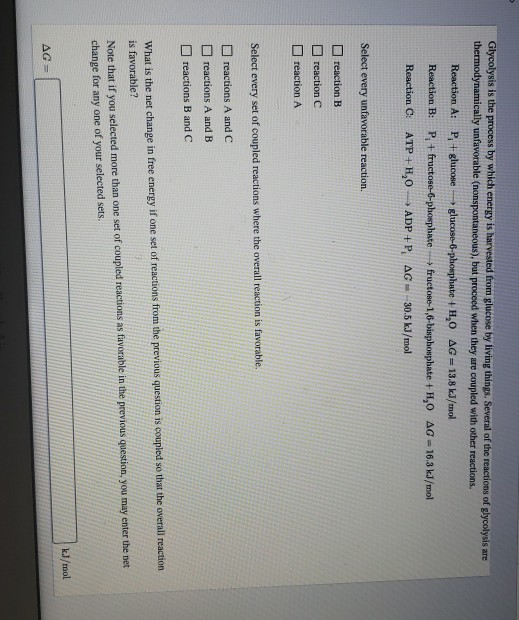Glycolysis is the process by which energy is harvested from glucose by living things. Several of the reactions of glycolysis are thermodynamically unfavorable (nonspontaneous), but proceed when they are coupled with other reactions. Reaction A: P + glucose glucose-6-phosphate +HO AG13.8 kJ/mol Reaction B: P fructose-6-phosphate + fructose-1,6-bisphosphate +1,0 AG 16.3 kJ/mol Reaction C: ATP+H, ADP +P, AG - 30.5 kJ/mol Select every unfavorable reaction. reaction B reaction C reaction A Select every set of coupled reactions where the overall...

• ### ignment Score: 69.4% Resources C Give Up? Feedback Try Again stion 7 of 12 > Attempt...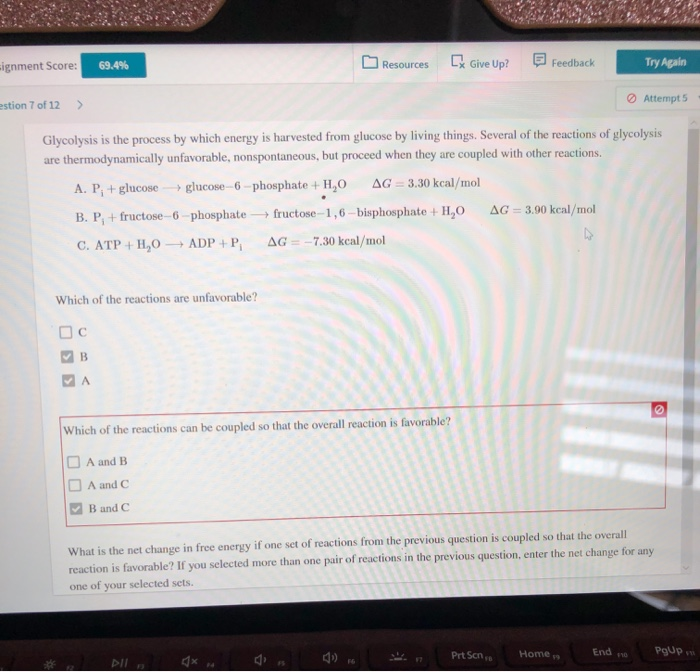ignment Score: 69.4% Resources C Give Up? Feedback Try Again stion 7 of 12 > Attempt 5 Glycolysis is the process by which energy is harvested from glucose by living things. Several of the reactions of glycolysis are thermodynamically unfavorable, nonspontaneous, but proceed when they are coupled with other reactions, A. P, + glucose glucose-6 phosphate + H,0 AG = 3.30 kcal/mol B. P, + fructose - 6 phosphate-fructose-1,6-bisphosphate + HO AG = 3.90 kcal/mol C. ATP+H,0 — ADP+P AG=...

• ### CHEM-1220: hemical Thermodynamics Coupled Reactions Resources « previous| 10 of 13 next >» Coupled Reactions Part...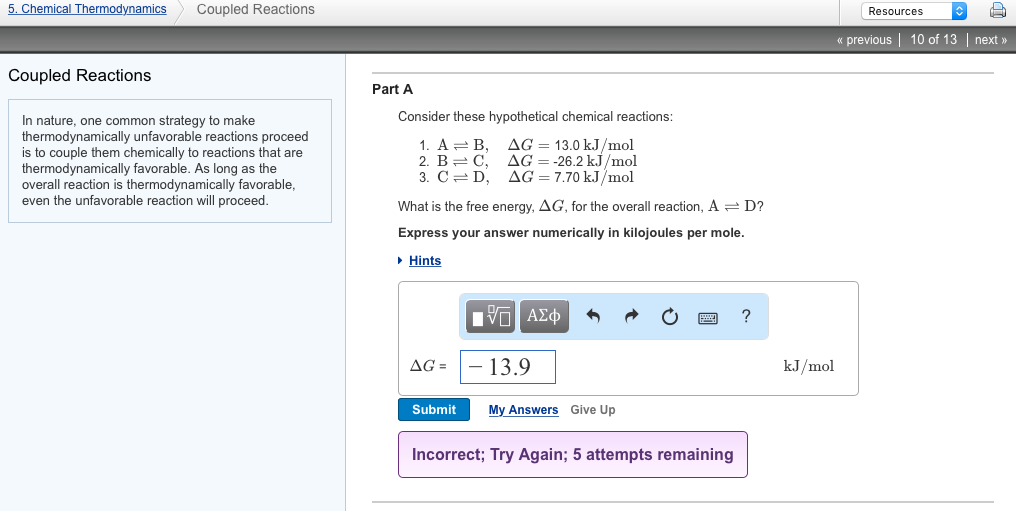CHEM-1220: hemical Thermodynamics Coupled Reactions Resources « previous| 10 of 13 next >» Coupled Reactions Part A Consider these hypothetical chemical reactions In nature, one common strategy to make thermodynamically unfavorable reactions proceed is to couple them chemically to reactions that are thermodynamically favorable. As long as the overall reaction is thermodynamically favorable even the unfavorable reaction will proceed 1. A-B, 2.B-C, 3, C D, ΔG= 13.0 kJ/mol ΔG=-26.2 kJ/mol ΔG= 7.70 kJ/mol What is the free energy, ΔG, for...

• ### In the first step of glycolysis, the given two reactions are coupled... thank you! In the...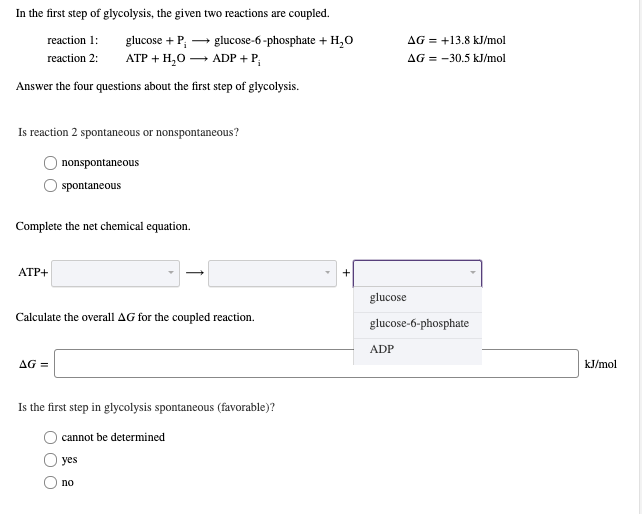In the first step of glycolysis, the given two reactions are coupled... thank you! In the first step of glycolysis, the given two reactions are coupled. reaction 1 glucose P АТР + H,О AG+13.8 kJ/mol AG 30.5 kJ/mol glucose-6-phosphate +H20 ADPP reaction 2: Answer the four questions about the first step of glycolysis Is reaction 2 spontaneous or nonspontaneous? nonspontaneous spontaneous Complete the net chemical equation АТР+ + glucose Calculate the overall AG for the coupled reaction. glucose-6-phosphate ADP AG...

• ### In the first step of glycolysis, the given two reactions are coupled. reaction 1: reaction 2:...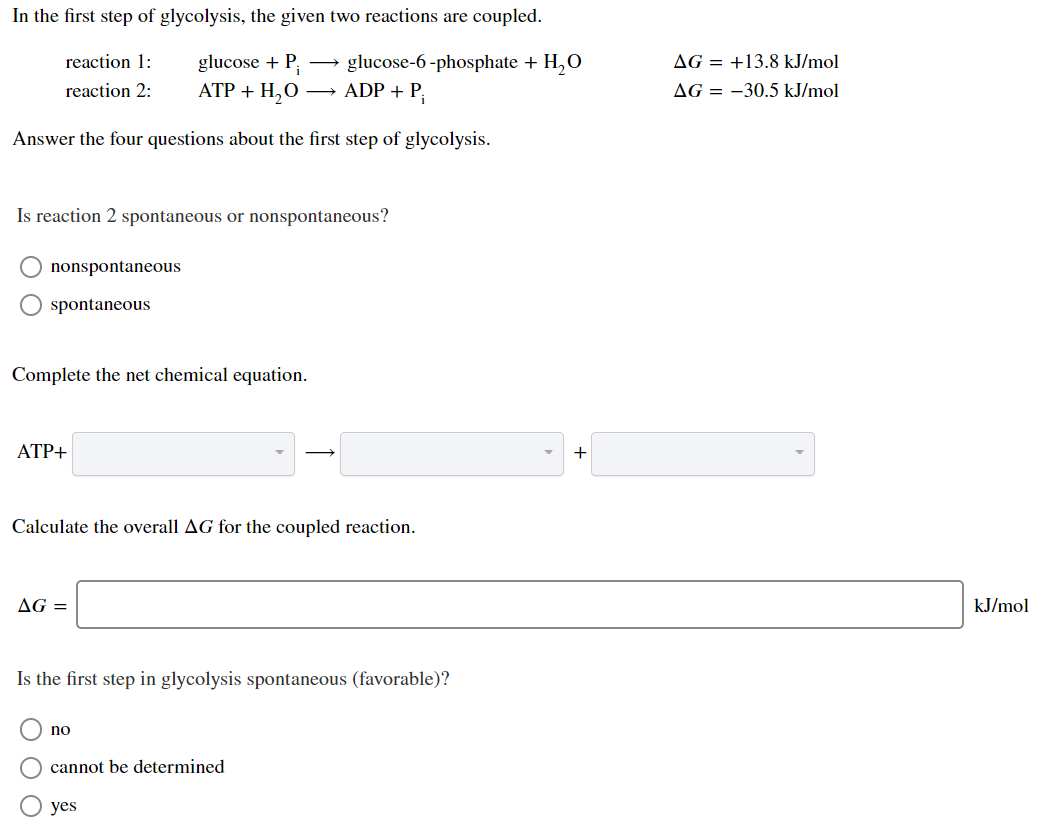In the first step of glycolysis, the given two reactions are coupled. reaction 1: reaction 2: glucose +P: ATP + H2O glucose-6-phosphate + H,O + ADP + P AG = +13.8 kJ/mol AG = -30.5 kJ/mol Answer the four questions about the first step of glycolysis. Is reaction 2 spontaneous or nonspontaneous? O nonspontaneous O spontaneous Complete the net chemical equation. ATP+ + Calculate the overall AG for the coupled reaction. AG = kJ/mol Is the first step in glycolysis...

• ### Glycolysis and Free Energy

The free energy for the oxidation of glucose to CO^2 and water is -686kcal/mol and the free energy for the reduction of NAD+ to NADH is +53 kcal/mol. Why are only twomolecules of NADH formed during glycolysis when it appears that as many as a dozen could be formed?A) There is no CO^2 or water produced during glycolysisB) Most of the free energy available from the oxidation of glucose is used in the production of ATP in glycolysisC) Most of...

• ### There are two sets of reactions in glycolysis in which phosphate intermediates are synthesized in...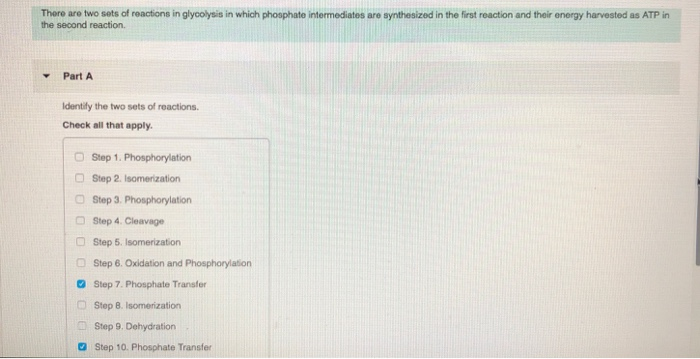There are two sets of reactions in glycolysis in which phosphate intermediates are synthesized in the first reaction and their energy harvested as ATP in the second reaction. Part A Identify the two sets of reactions. Check all that apply. O Step 1. Phosphorylation Step 2. Isomenzation Step 3. Phosphorylation Step 4. Cleavage Step 5, Isomerization Step 6, Oxidation and Phosphorylaton Step 7. Phosphate Transfer Step 8. Isomerization Step 9, Dehydration C) □ a Step 10. Phosphate Transfer We were...

• ### 1. In a cell, a thermodynamically unfavorable process can occur if the process is coupled to...

1. In a cell, a thermodynamically unfavorable process can occur if the process is coupled to a thermodynamically favorable process. the process is thermodynamically favorable outside the cell. an enzyme gives energy to the reacting substances. the cell as a whole is close to equilibrium. 2. In an active transport protein, ATP hydrolysis creates a channel through the membrane. ATP uses the protein to move across the membrane. ATP hydrolysis causes the protein to change shape. ATP carries a substance...

• ### 3. Summary of glycolysis Aa Aa E The removal of high-energy electrons from glucose begins with...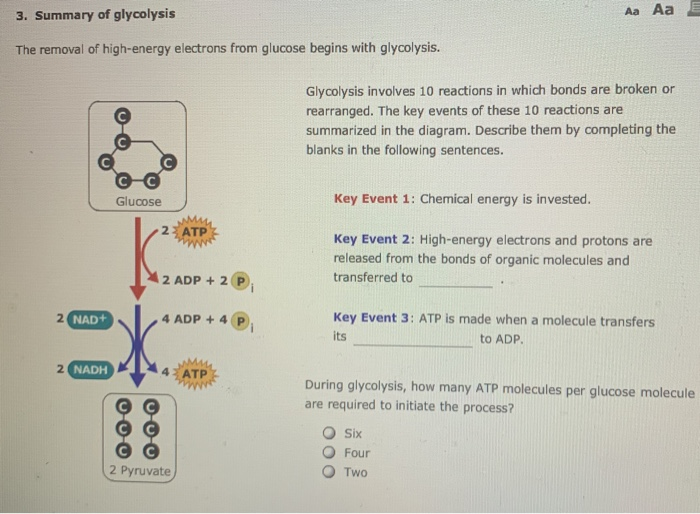3. Summary of glycolysis Aa Aa E The removal of high-energy electrons from glucose begins with glycolysis. Glycolysis involves 10 reactions in which bonds are broken or rearranged. The key events of these 10 reactions are summarized in the diagram. Describe them by completing the blanks in the following sentences. Glucose Key Event 1: Chemical energy is invested. Key Event 2: High-energy electrons and protons are released from the bonds of organic molecules and transferred to 2 ADP + 2...

• ### Living organisms use energy from the metabolism of food to create an energy rich molecule called...

Living organisms use energy from the metabolism of food to create an energy rich molecule called adenosine triphoshpate (ATP). the ATP then acrs as and energy source for a variegty of reactions that the living organism must carry oyut to survive. ATP provides energy through its hydrolysis, which can be symbolized as follows: ATP(aq)+H2O(l) --> ADP(aq)+Pi(aq) with a delta G of the reaction being =-30.5 kj. Where ADP represents adenosine diphosphate and Pi represents in inorganic phosphate group (such as...